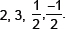Polynomials-Notes

NOTES FOR POLYNOMIALS
Basic Concepts
Zeroes of a polynomial. k is said to be zero of a polynomial p(x) if p(k) = 0
Graph of polynomial.
(i)   Graph of a linear polynomial ax + b is a straight line.
(ii)   Graph of a quadratic polynomial p(x) = ax2 + bx + c is a parabola open upwards like ∪, if a > 0.
(iii)   Graph of a quadratic polynomial p(x) = ax2 + bx + c is a parabola open downwards like ∩, if a > 0.
(iv)   In general a polynomial p(x) of degree n crosses the x-axis at atmost n points.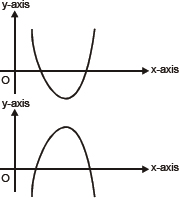Relationship between the zeroes and the coefficients of a Polynomial.
(i)   If α, β are zeroes / roots of p(x) = ax2 + bx + c, then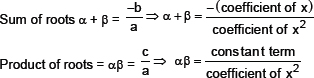(ii)   If α, β and γ are zeroes / roots of p(x) = ax3 + bx2 + cx + d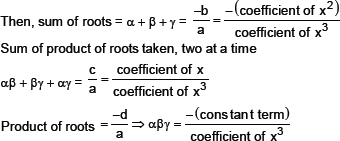(iii)   If α, β are roots of a quadratic polynomial p(x), then p(x) = x2 – (α + β) x + αβ
⇒ p(x) = x2 – (sum of roots) x + product of roots
(iv)   If α, β and γ are zeroes of a cubic polynomial p(x),
Then, p(x) = x3 – (α + β + γ) x2 + (αβ + βγ + αγ) x – (αβγ)
⇒ p(x) = x3 – (sum of zeroes) x2 + (sum of product of zeroes / roots taken two at a time)
x – (product of zeroes)
1.  Find the zeroes of the quadratic polynomial and verify the relationship between the zeroes and coefficient of polynomial p(x) = x2 + 7x + 12.
Sol. p(x) = x2 + 7x + 12
⇒ p(x) = (x + 3)(x + 4)
∴ p(x) = 0 if x + 3 = 0 or x + 4 = 0
⇒ x = – 3 or x = – 4
∴– 3 and – 4 are zeros of the p(x).
Now,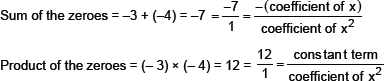2.  Find the zeroes of 4x2 – 7 and verify the relationship between the zeroes and its coefficients.
Sol.  Let p(x) = 4x2 – 7
Here coefficient of x2 = 4,
Coefficient of x = 0 and constant term = –7.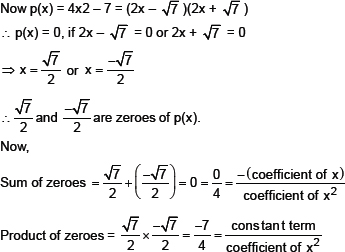3.  Find a quadratic polynomial whose zeroes are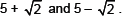Sol.  Let α, β are zeroes of quadratic polynomial p(x).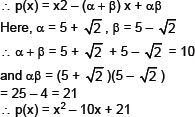4.  Find a quadratic polynomial, the sum of whose zeroes is 0 and one zero is 5.
Sol.  Let zeroes are α and β.
⇒α + β = Sum of zeroes
⇒α + β = 0 ⇒ 5 + β = 0 ⇒β = –5
Now product of zeroes = αβ = 5 × (–5) = –25
Let polynomial p(x) = ax2 + bx + c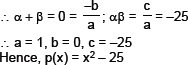5.  Find a cubic polynomial with the sum, sum of the product of its zeroes taken two at a time, and product of its zeroes are 5, –6 and –20 respectively.
Sol.  Let p(x) = ax3 + bx2 +cx + d
and &alpha, &beta, γ are its zeroes.
∴ α + β + γ = Sum of zeroes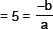αβ + αγ + βγ = Sum of the products of zeroes taken two at a time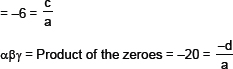If a = 1, then b = –5, c = –6 and d = 20
∴ Polynomial, p(x) = x3 – 5x2 – 6x + 20.
Division Algorithm for polynomials.
If p(x) and g(x) are any two polynomials with g(x) ≠ 0, then we can find polynomials q(x) and r(x) such that
p(x) = q(x) × g(x) + r(x), where r(x) = 0 or degree of r(x) < degree of g(x).
Step–1. Divide the highest degree term of the dividend by the highest degree term of the divisor and obtain the remainder.
Step2. If the remainder is 0 or degree of remainder is less than divisor, then we cannot continue the division any further. If degree of remainder is equal to or more than divisor repeat step-1.
6.  Divide 4x3 + 2x2 + 5x – 6 by 2x2 + 3x + 1.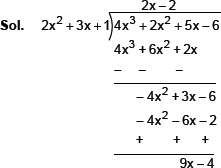∴ Quotient is 2x – 2 and remainder is 9x – 4.
7.  Find all zeroes of polynomial
4x4 – 20x3 + 23x2 + 5x – 6 if two of its zeroes are 2 and 3.
Sol. Since two zeroes are 2 and 3.
∴ (x – 2)(x – 3) is a factor of given polynomial.
⇒ x2 – 5x + 6 is a factor of given polynomial.
Now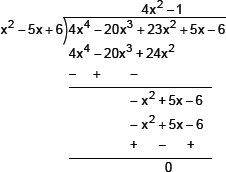∴ 4x4 – 20x3 + 23x2 + 5x – 6
= (x2 – 5x + 6)(4x2 – 1)
= (x – 2)(x – 3)(2x – 1)(2x + 1)
∴ Zeroes of the given polynomial are